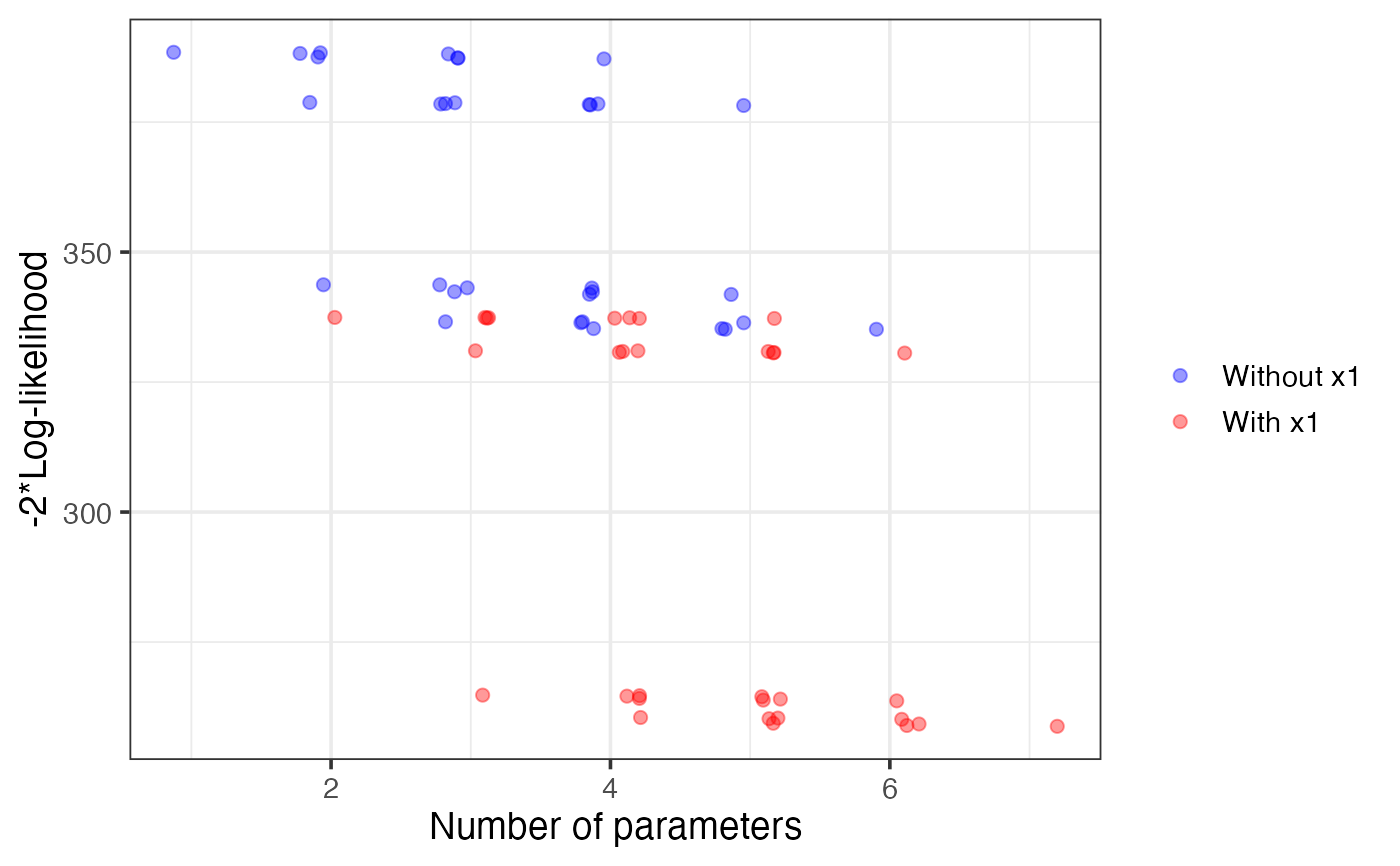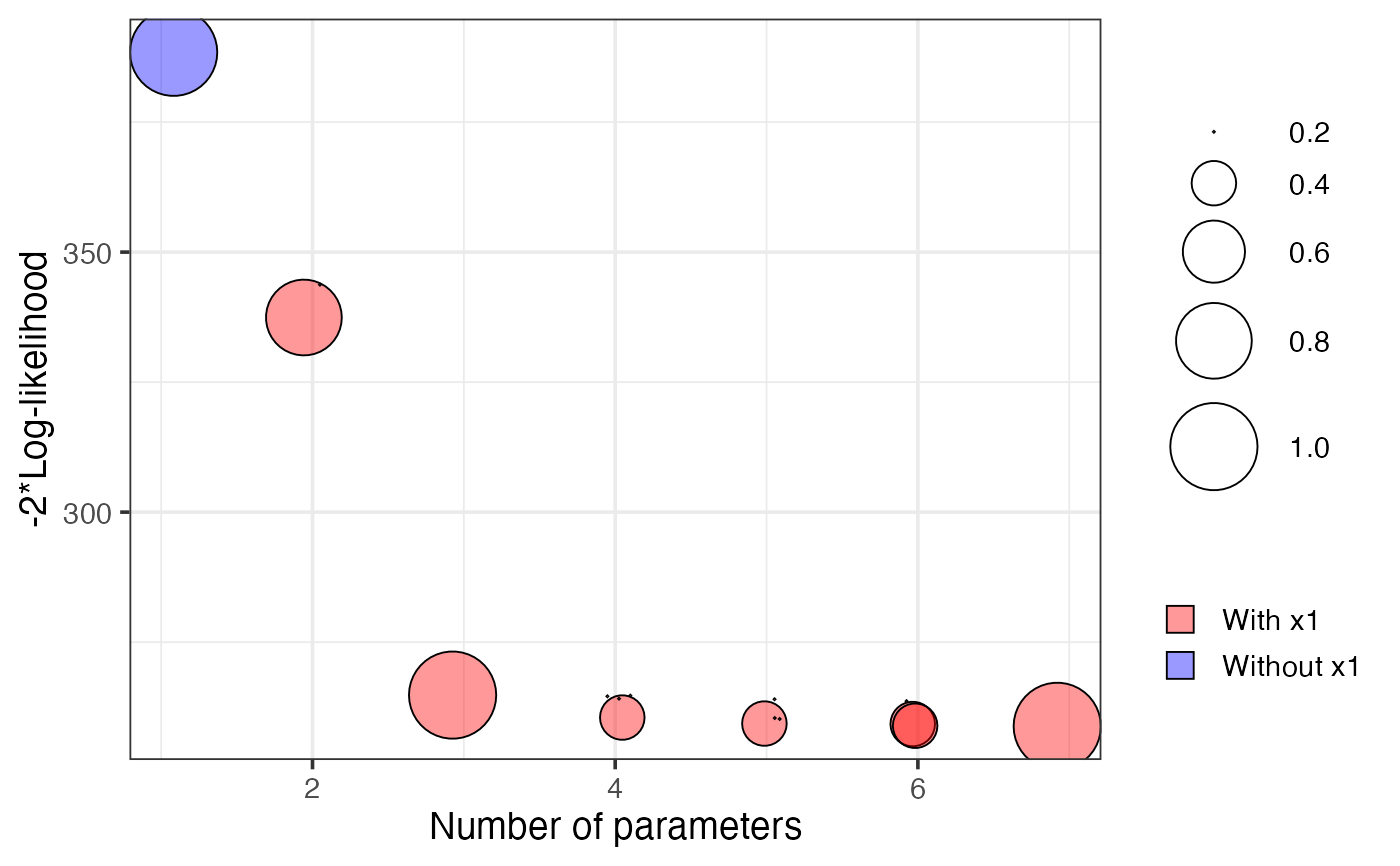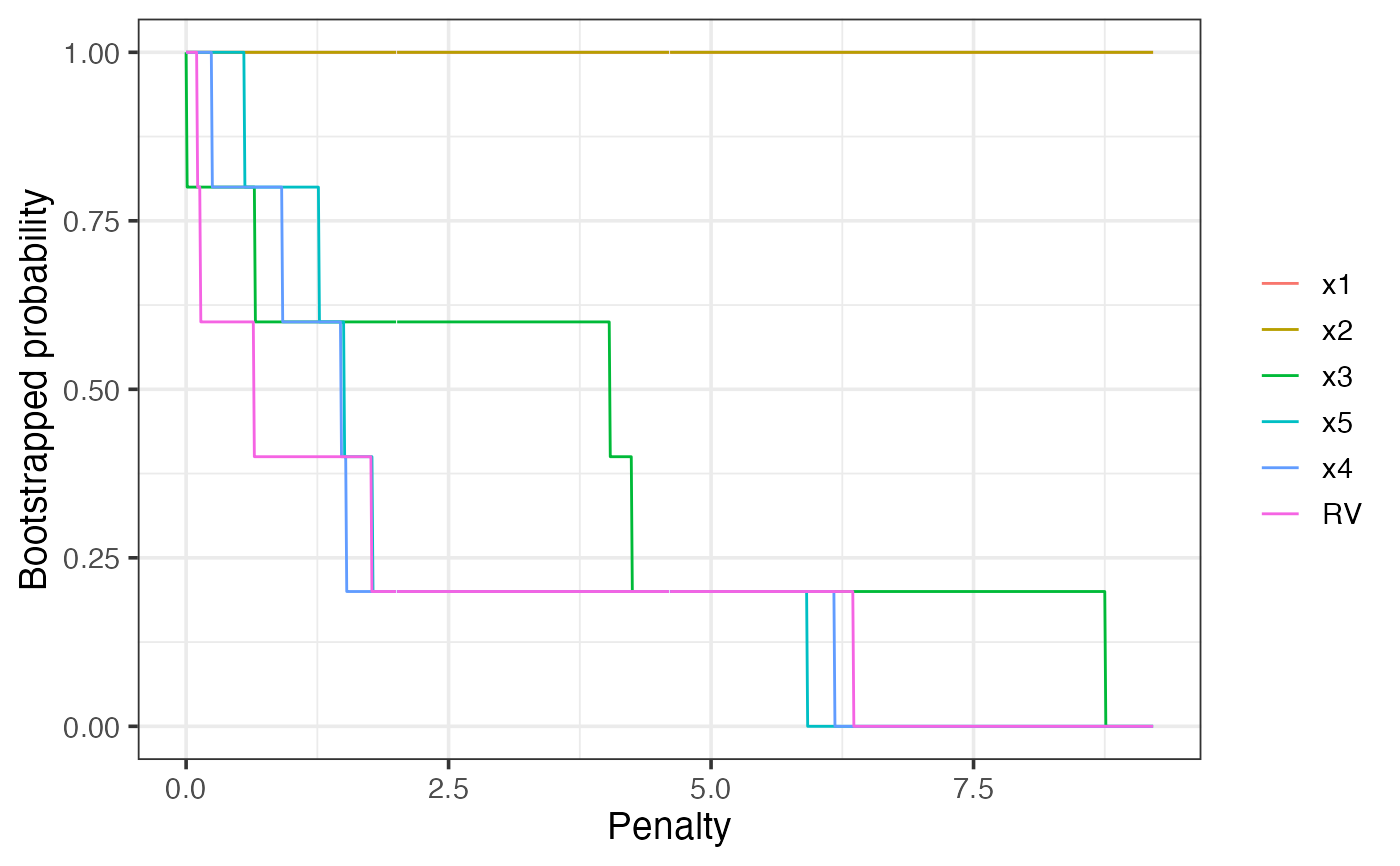Calculates and provides the plot methods for standard and bootstrap enhanced model stability plots (lvk and boot) as well as variable inclusion plots (vip).

vis(
mf,
nvmax,
B = 100,
lambda.max,
nbest = "all",
use.glmulti = FALSE,
cores,
force.in = NULL,
screen = FALSE,
redundant = TRUE,
seed = NULL,
...
)

## Arguments

mf a fitted 'full' model, the result of a call to lm or glm (and in the future lme or lmer) size of the largest model that can still be considered as a viable candidate number of bootstrap replications maximum penalty value for the vip plot, defaults to 2*log(n) maximum number of models at each model size that will be considered for the lvk plot. Can also take a value of "all" which displays all models. logical. Whether to use the glmulti package instead of bestglm. Default use.glmulti=FALSE. number of cores to be used when parallel processing the bootstrap the names of variables that should be forced into all estimated models. (Not yet implemented.) logical, whether or not to perform an initial screen for outliers. Highly experimental, use at own risk. Default = FALSE. logical, whether or not to add a redundant variable. Default = TRUE. random seed for reproducible results further arguments (currently unused)

## Details

The result of this function is essentially just a list. The supplied plot method provides a way to visualise the results.

See ?plot.vis or help("plot.vis") for details of the plot method associated with the result.

Mueller, S. and Welsh, A. H. (2010), On model selection curves. International Statistical Review, 78:240-256. doi: 10.1111/j.1751-5823.2010.00108.x

Murray, K., Heritier, S. and Mueller, S. (2013), Graphical tools for model selection in generalized linear models. Statistics in Medicine, 32:4438-4451. doi: 10.1002/sim.5855

Tarr G, Mueller S and Welsh AH (2018). mplot: An R Package for Graphical Model Stability and Variable Selection Procedures. Journal of Statistical Software, 83(9), pp. 1-28. doi: 10.18637/jss.v083.i09

plot.vis

## Examples

n = 100
set.seed(11)
e = rnorm(n)
x1 = rnorm(n)
x2 = rnorm(n)
x3 = x1^2
x4 = x2^2
x5 = x1*x2
y = 1 + x1 + x2 + e
dat = data.frame(y, x1, x2, x3, x4, x5)
lm1 = lm(y ~ ., data = dat)
# \dontshow{
v1 = vis(lm1, B = 5, cores = 1, seed = 1)
plot(v1, highlight = "x1", which = "lvk")plot(v1, which = "boot")plot(v1, which = "vip")# }
if (FALSE) {
v1 = vis(lm1, seed = 1)
plot(v1, highlight = "x1", which = "lvk")
plot(v1, which = "boot")
plot(v1, which = "vip")
}